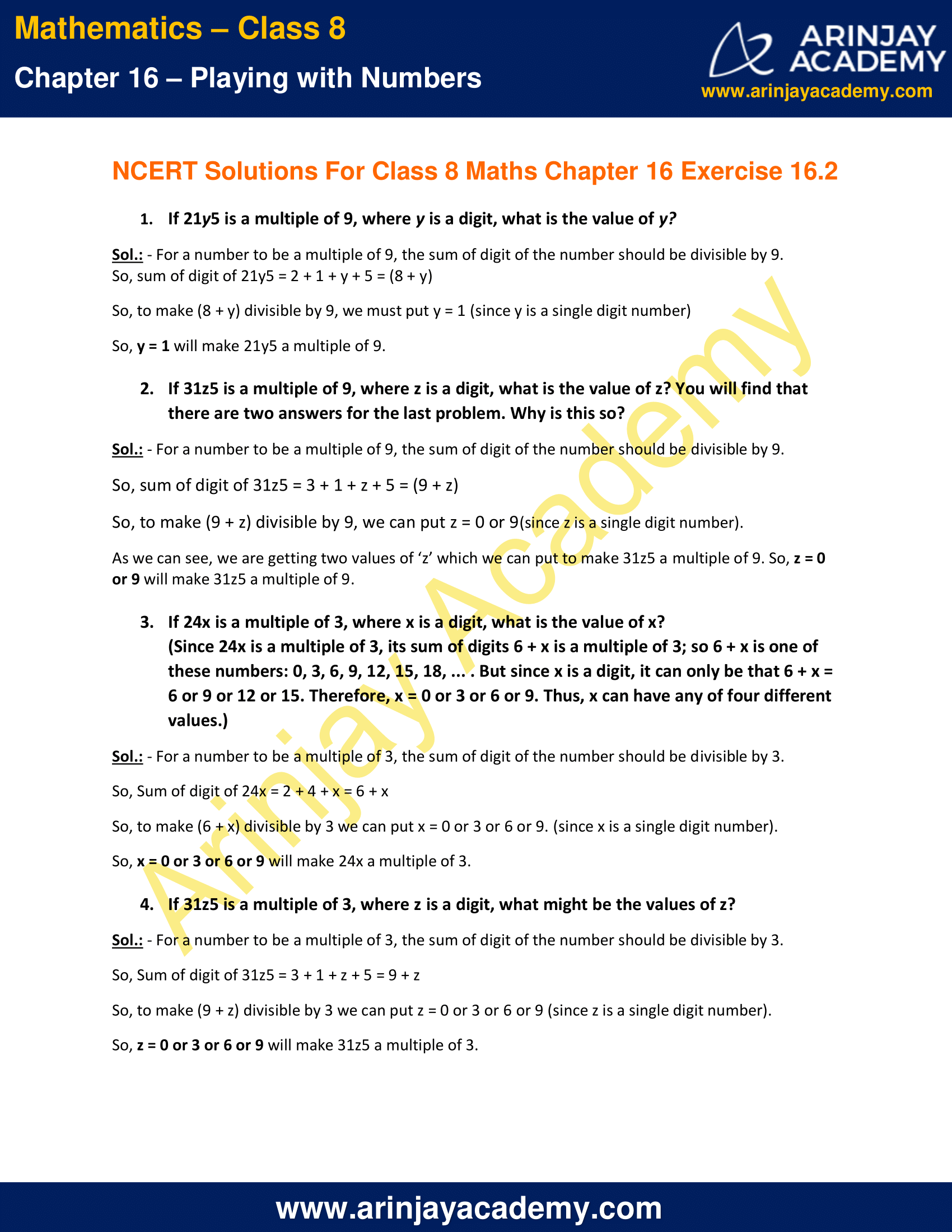# NCERT Solutions for Class 8 Maths Chapter 16 Exercise 16.2 – Playing with Numbers

Download NCERT Solutions for Class 8 Maths Chapter 16 Exercise 16.2 – Playing with Numbers. This Exercise contains 4 questions, for which detailed answers have been provided in this note. In case you are looking at studying the remaining Exercise for Class 8 for Maths NCERT solutions for Chapter 16 or other Chapters, you can click the link at the end of this Note.

### NCERT Solutions for Class 8 Maths Chapter 16 Exercise 16.2 – Playing with NumbersNCERT Solutions for Class 8 Maths Chapter 16 Exercise 16.2 – Playing with Numbers

1. If 21y5 is a multiple of 9, where y is a digit, what is the value of y?

Sol.: – For a number to be a multiple of 9, the sum of digit of the number should be divisible by 9.
So, sum of digit of 21y5 = 2 + 1 + y + 5 = (8 + y)
So, to make (8 + y) divisible by 9, we must put y = 1 (since y is a single digit number)
So, y = 1 will make 21y5 a multiple of 9.

2. If 31z5 is a multiple of 9, where z is a digit, what is the value of z? You will find that there are two answers for the last problem. Why is this so?

Sol.: – For a number to be a multiple of 9, the sum of digit of the number should be divisible by 9.
So, sum of digit of 31z5 = 3 + 1 + z + 5 = (9 + z)
So, to make (9 + z) divisible by 9, we can put z = 0 or 9(since z is a single digit number).
As we can see, we are getting two values of ‘z’ which we can put to make 31z5 a multiple of 9. So, z = 0 or 9 will make 31z5 a multiple of 9.

3. If 24x is a multiple of 3, where x is a digit, what is the value of x?
(Since 24x is a multiple of 3, its sum of digits 6 + x is a multiple of 3; so 6 + x is one of these numbers: 0, 3, 6, 9, 12, 15, 18, … . But since x is a digit, it can only be that 6 + x = 6 or 9 or 12 or 15. Therefore, x = 0 or 3 or 6 or 9. Thus, x can have any of four different values.)

Sol.: – For a number to be a multiple of 3, the sum of digit of the number should be divisible by 3.
So, Sum of digit of 24x = 2 + 4 + x = 6 + x
So, to make (6 + x) divisible by 3 we can put x = 0 or 3 or 6 or 9. (since x is a single digit number).
So, x = 0 or 3 or 6 or 9 will make 24x a multiple of 3.

4. If 31z5 is a multiple of 3, where z is a digit, what might be the values of z?

Sol.: – For a number to be a multiple of 3, the sum of digit of the number should be divisible by 3.
So, Sum of digit of 31z5 = 3 + 1 + z + 5 = 9 + z
So, to make (9 + z) divisible by 3 we can put z = 0 or 3 or 6 or 9 (since z is a single digit number).
So, z = 0 or 3 or 6 or 9 will make 31z5 a multiple of 3.

The next Exercise for NCERT Solutions for Class 8 Maths Chapter 16 Exercise 16.1 – Playing with Numbers can be accessed by clicking here

Download NCERT Solutions for Class 8 Maths Chapter 16 Exercise 16.2 – Playing with Numbers